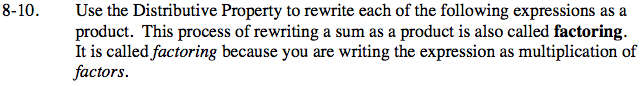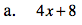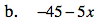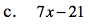Home > ACC7 > Chapter cc38 > Lesson cc38.1.1 > Problem8-10

8-10.
1. Use the Distributive Property to rewrite each of the following expressions as a product. This process of rewriting a sum as a product is also called factoring. It is called factoring because you are writing the expression as multiplication of factors. Homework Help ✎

1. 4x + 8

2. −45 − 5x

3. 7x − 21What value can you factor out of this expression?

Factor out a 4 and rewrite the expression.

4(x + 2)See part (a).

−5(9 + x)See part (a).